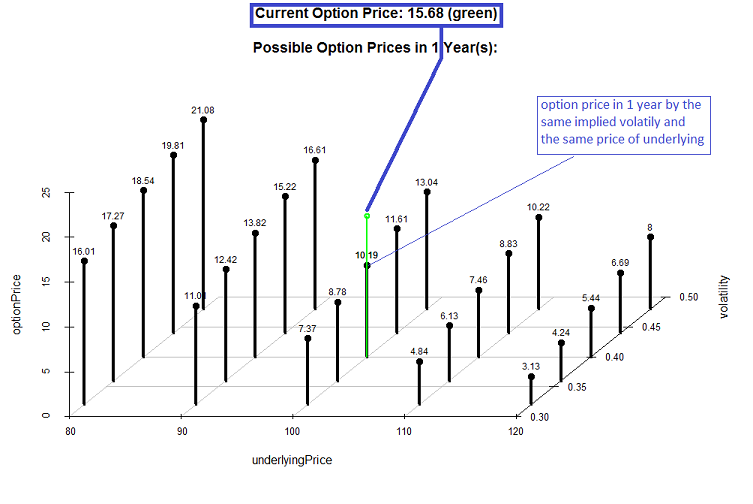# Online Option Calculator – estimate the future value of an option

Many retail investors are unaware that the option price sometimes depends on the (implied) volatility much stronger than on the price of underlying. They also often underestimate the losses of time value. Our option calculator lets you estimate the future fair price of an option by different pairs of implied volatility and underlying price.We support the calculation of American and European CALLs and PUTs. We also take dividends into accounts. Dividends increase the value of a PUT and decrease the value of a CALL option. Note that if you use the implied volatility, the dividend-yield is usually already taken into consideration, so set it to zero. But never neglect it if you calculate with historical volatility, esp. in case of a stock, which is known for its generous dividend payoffs.

 Option Type CALL PUT Option Style American European Current Price of Underlying Strike Maturity (in years) Risk-free Rate (implied) Volatility Dividend-Yield Planning Horizon (in years)
Chart Settings (modify only if you get an ugly chart)

range underlying price (0.1 the narrowest and 0.99 the widest)

range volatility (0.01 the narrowest and 0.9 the widest)

view angle (how to turn the 3d chart)

[collapse]

Like this post and wanna learn more? Have a look at Knowledge rather than Hope: A Book for Retail Investors and Mathematical Finance Students

FinViz - an advanced stock screener (both for technical and fundamental traders)